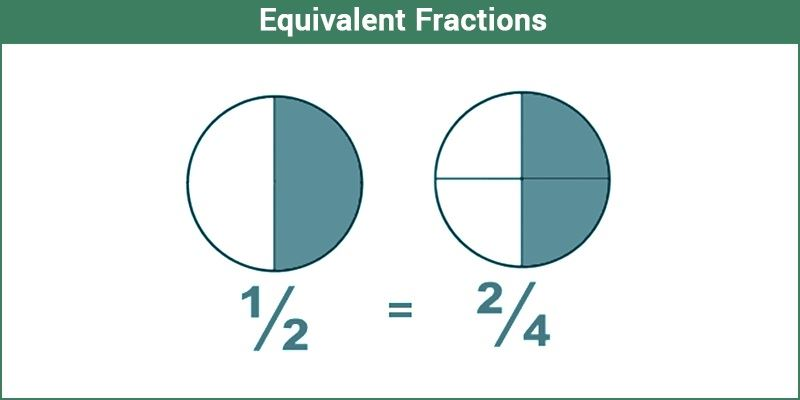Equivalent Fractions

The equivalent fractions are a type of fraction which seems to be different (not having the exact numbers) but they are equivalent in nature(having exactly equal value). Equivalent refers to being equal in value, function, amount or meaning and such things. For example, an example of the equivalent fraction is- 3/9 = 13/39 =1/3. These are the fractions which have equal values both in numerator and denominator after simplification. Let us understand with the help of definition and solved examples here in this article.

Equivalent Fractions Definition

The definition of equivalent fractions states that two or more than two fractions are said to be equal if both results in same fraction after simplification. Let us say, a/b and c/d are two fractions, if after simplification they both result in equivalent fraction, say e/f, then they are equal to each other.The biggest question here can be, why do they have equal values in spite of having different number?

The answer to this question is that, as the numerator and denominator are not co-prime numbers, therefore they have a common multiple which on division given an exactly the same value.

Take for an example:

1/2 = 2/4 = 4/8

But, it is clearly seen that the above fractions have different numbers as numerators and denominators.

Dividing both numerator and denominator by their common factor, we have:

$\large \frac{4 \div 4}{8 \div 4}$

=1/2

In the same way, if we simplify 2/4, again get 1/2.

$\large \frac{2\div 2}{4\div 2}$

= 1/2

How to Find Equivalent Fractions?

These fractions are actually the same because when we multiply or divide both, the numerator and the denominator by the same number, the value of the fraction actually doesn’t change. Therefore, equivalent fractions when reduced to their simplified value will all be the same.

For example, consider the fraction 1/5

Multiplying numerator and denominator with 2, we get 1/5 = 2/10

Multiplying numerator and denominator with 3, we get 1/5 = 3/15

Multiplying numerator and denominator with 4, we get 1/5 = 4/20

Therefore, we can conclude that,

1/5 = 2/10 = 3/15 = 4/20

Keep in mind that, we can only multiply or divide by the same numbers to get an equivalent fraction, and not add or subtract. Simplification to get equivalent numbers can be done till a point where both, the numerator and denominator should still be whole numbers.

Try the following question to check your understanding of the concept of equivalent fractions.

Equivalent Fractions Examples

Example 1: The given fractions 5/16 and x/12 are equivalent fractions, then find the value of x.

Solution:

Given: 5/16 = x/12

x = (5 x 12)/6

x = 10

Example 2: Two fractions 3/5 and 4/x are equivalent. Find the value of x.

Solution: Given,

3/5 = 4/x

x = (4 x 5)/3

x = 20/3

Have persisting doubts on any topic? Try reaching out to our mentors only with BYJU’s – The Learning App.